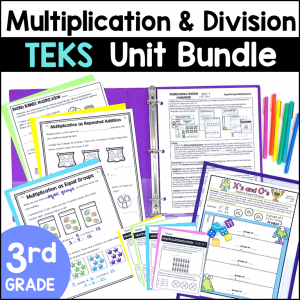Save 10% on your first order with code EXTRA10

# No Prep Math Journal Prompts – 3rd Grade Multiplication and Division TEKS

\$3.00\$29.99
SKU: 8596348 Category:

## Description

Need high quality, No Prep Multiplication and Division Activities? You will love this set of Math Journal Prompts that encourage deep thinking and help you collect data on student learning. Students will enjoy drawing and writing about multiplication and division word problems, math facts, arrays, models and much more!

These journal prompts give students an opportunity to think deeply about concepts covered in the TEKS and to see patterns.

I absolutely love using math journals in the classroom! They are the perfect way to engage students in deep thinking and assess their understanding. Math journal prompts are naturally differentiated, as students are able to dive as deep into content as they are able to.

What’s Included:

• 22 Journal Prompts
• 10 Full page Journal Prompt Responses that can be used as examples at a math station.
• Teaching Tips for using the journal prompts

Great ways to use these journal prompts:

• No-Prep Math Station
• Homework (that isn’t busy work)
• Guide a Small Group Lesson
• Tutoring Sessions
• Review
• Collect Qualitative Data for Parent-Teacher Conferences or PLC and RTI Meetings

Topics include:

• One-step Multiplication and Division Word Problems
• Arrays
• Representing Multiplication with Repeated Addition, Skip Counting, Jumps on a Number Line, Area Models and Arrays
• Relationships between Multiplication and Division
• Division of objects into Equal Groups or taking out Equal Sets
• Odd and Even Numbers
• Describing Multiplication as a Comparison
• Finding Missing Numbers in Multiplication and Division equations

TEKS Alignment:

3.4D: Determine the total number of objects when equally sized groups of objects are combined or arranged in arrays up to 10 by 10.

3.4E: Represent multiplication facts by using a variety of approaches such as repeated addition, equal-sized groups, arrays, area models, equal jumps on a number line, and skip counting.

3.4F: Recall facts to multiply up to 10 by 10 with automaticity and recall the corresponding division facts.

3.4H: Determine the number of objects in each group when a set of objects is partitioned into equal shares or a set of objects is shared equally.

3.4I: Determine if a number is even or odd using divisibility rules.

3.4J: Determine a quotient using the relationship between multiplication and division.

3.5C: Describe a multiplication expression as a comparison such as 3 x 24 represents 3 times as much as 24.

3.5D: Determine the unknown whole number in a multiplication or division equation relating three whole numbers when the unknown is either a missing factor or product.